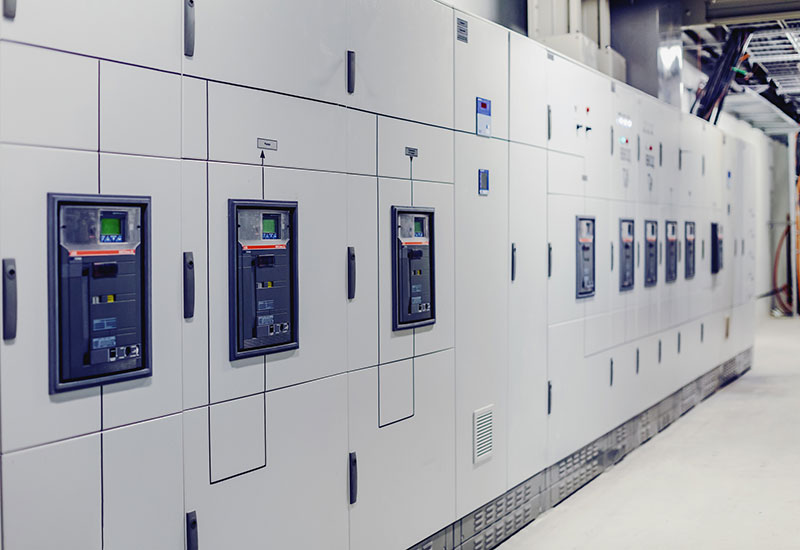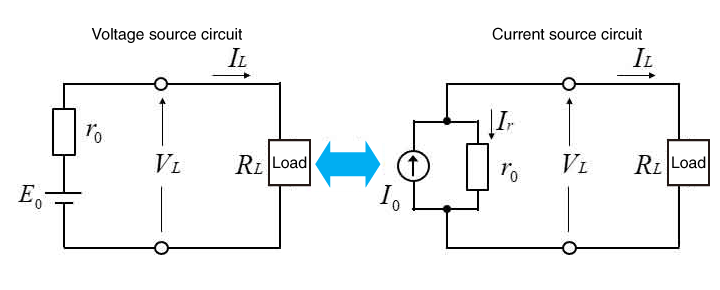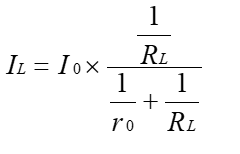Searching...

# Tech Tips## About Constant Voltage (CV) Power Supply and Constant Current (CC) Power Supply

A power supply is a device that supplies electrical energy to one or more electric loads. They are primarily of two types: voltage sources and current sources. In general, "power supply" often means a voltage source, but there are also current sources that supply constant current.
A power circuit that converts incoming electricity into the required form and outputs it is called a "power circuit." Power supply circuits can be roughly divided into "constant-voltage power supply" and "constant-current power supply."

### Constant-voltage power supply

A constant-voltage power supply is a power circuit that controls the output voltage to a constant level. It always supplies a constant voltage regardless of the load and is widely used in power supplies for electronic circuits.
Most electronic circuits are designed to operate at a constant voltage because they cannot handle unexpected voltage fluctuations.

### Constant-current power supply

A constant-current power supply is a power supply circuit that controls the output current to a constant level. Constant-current power supplies are used to power LED lighting and charge rechargeable batteries.
The brightness of LED lighting is determined by the current value; therefore, if the current value fluctuates, the brightness changes accordingly. Although not an issue for small LED screens, the change in brightness is visible for large lights and a stable current is therefore required.
Also, when charging rechargeable batteries, the voltage and current are not proportional due to the characteristics of rechargeable batteries. Therefore, a constant current power supply is employed so that the current is supplied regardless of the voltage applied to the battery.

### Conversion between the voltage source and current source

Voltage and current sources can be converted to each other equivalently. When the voltage applied to the load and the current flowing through the load are the same, the voltage source and the current source operate as having the same value and function.
In other words, a voltage source can be equivalently converted into a current source that does the same work for the load, and vice versa.### Variable Switching Power Supply

Some power supplies are "variable switching power supplies" that can switch modes to enable various outputs. In addition to constant voltage (CV) mode and constant current (CC) mode, modes such as constant power (CP) and constant resistance (CR) modes are available to provide output according to the application.

## Principle of stabilization

The circuit of a constant voltage source is shown below. The voltage source contains a power supply and internal resistors, which are connected in series with the power supply. The terminal voltage VL is expressed as follows:

#### r0＋RL

Therefore, when the internal resistance r0 is sufficiently small compared to the load resistance RL, VL≒E0. This results in a constant terminal voltage VL regardless of the load current IL.The circuit of a constant-current power supply is as shown below. The internal resistance of the constant-current power supply is in parallel with the power supply. The output current IL is expressed as follows:When the internal resistance r0 is ∞, IL≒I0. This makes the load current IL constant.A power supply that has a mechanism of maintaining VL and IL constant is called a DC stabilized power supply.
The types of DC-regulated power supplies include "series regulated power supplies," in which a control circuit is inserted between the input and output to stabilize the voltage and current, and "switching power supplies," in which high frequency is created by turning the input off and on, and then rectified and controlled for stabilizing.
DC-regulated power supplies are broadly classified into "DC constant voltage power supplies" whose output voltage is stable even when the load changes and "DC constant current power supplies" whose output current is stable.
Programmable DC power supplies automatically switch to constant voltage (CV) mode or constant current (CC) mode, depending on the voltage and current settings and the load connected.
For more information on the merits and demerits of DC power supplies depending on the control method and the devices they handle, please refer to the links below.